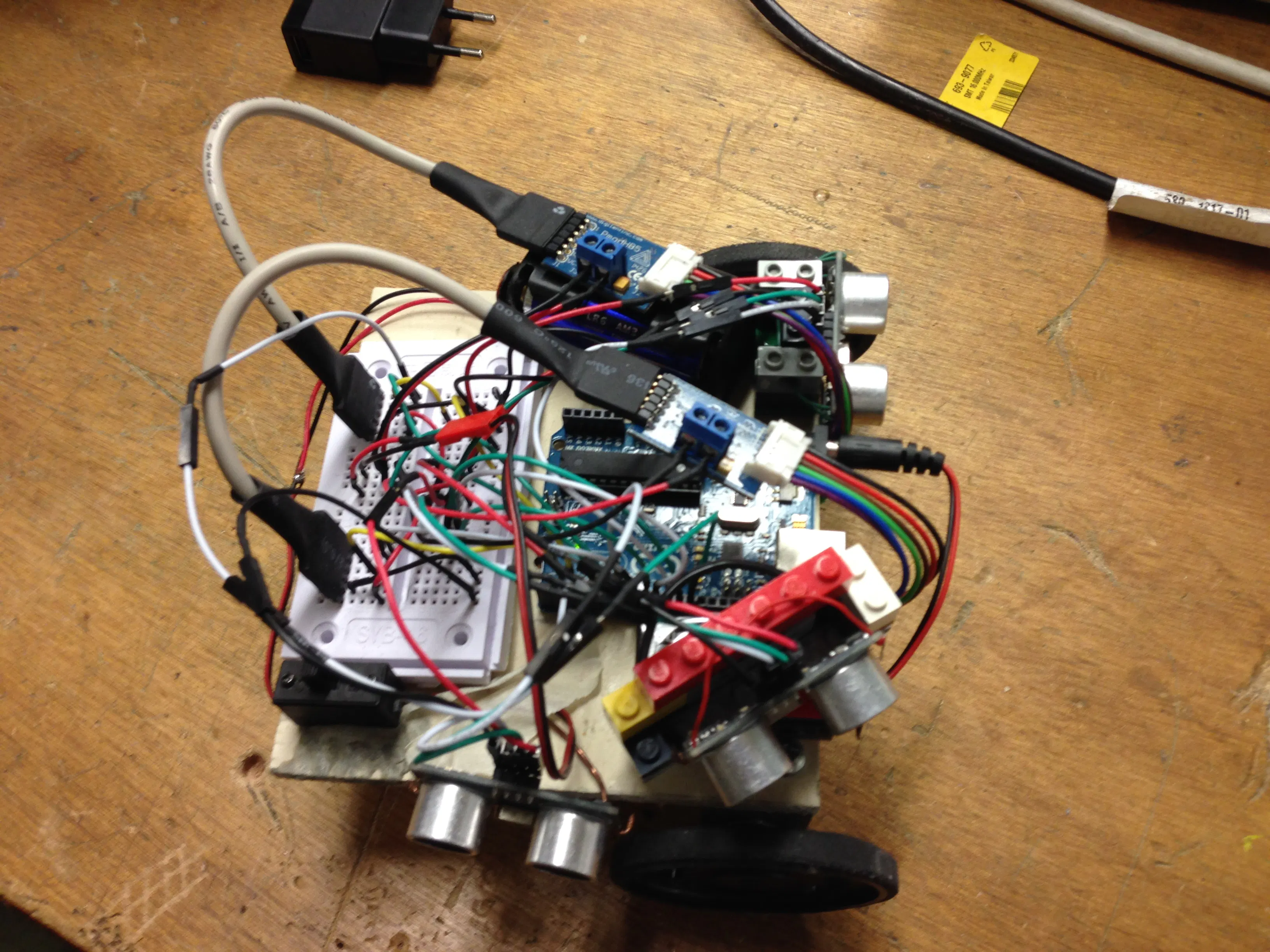Published

# Maze Robot

This robot is able to solve a maze.

IntermediateShowcase (no instructions)873## Things used in this project

### Hardware componentsArduino UNO & Genuino UNO
×1×1Jumper wires (generic)
×1

## Code

### robot maze code

Arduino
```#include <NewPing.h>
#include <SimpleTimer.h>// importation d'un timer

#define SONAR_NUM     3 // Number or sensors.
#define MAX_DISTANCE 200 // Maximum distance (in cm) to ping.
#define PING_INTERVAL 33 // Milliseconds between sensor pings (29ms is about the min to avoid cross-sensor echo).

// initialisation des pin et des variable
SimpleTimer timer;
// pin d'alimentation pont en h peut etre remplacer par la borne 5V de l'arduino
int capteur_moteur1 = 2; // pin du capteur moteur 1
int Enable_moteur1 = 6; // pin de commande de vitesse du moteur 1
int Direction_moteur1 = 8; // pin de commande de direction 1
int capteur_moteur2 = 3; // pin du capteur moteur 2
int Enable_moteur2 = 5; // pin commande vitesse du moteur 2
int Direction_moteur2 = 4; // pin de direction du moteur 2
unsigned int tick_codeuse_m2; // compteur d'impulsion du moteur 2
unsigned int tick_codeuse_m1; // compteur d'impulsion du moteur 1
unsigned long pingTimer[SONAR_NUM]; // Holds the times when the next ping should happen for each sensor.
unsigned int cm[SONAR_NUM];         // Where the ping distances are stored.
uint8_t currentSensor = 0;          // Keeps track of which sensor is active.

// donnee du moteur
float rapport_reducteur = 53;
int tick_tour_codeuse = 6; // nombre d'impulsion par tours des moteur

// variable de l'asservissement
const int frequence_echantillonnage = 50; // frequence du calcul de vitesse
int volatile cmd1;
int volatile cmd2;

// variable de la correction PID
float kp = 150; //150
float ki = 0.1;
float kd = 50;
float ki2 = 800;
float erreur_precedente_m1;
float erreur_precedente_m2;
float erreur_precedente;
float distance_mur_devant;
float distance_mur_45;
float distance_mur_droite;
int erreur;
int consigne=20;
//int cmd = 255;
unsigned long tempo = 0;
int nvariable = 0;

NewPing sonar[SONAR_NUM] = {     // Sensor object array.
NewPing(12, 11, MAX_DISTANCE), // Each sensor's trigger pin, echo pin, and max distance to ping.
NewPing(9, 10, 45),
NewPing(13, 7, 200),
};

void setup() {
Serial.begin(115200);
pingTimer = millis() + 75;           // First ping starts at 75ms, gives time for the Arduino to chill before starting.
for (uint8_t i = 1; i < SONAR_NUM; i++) // Set the starting time for each sensor.
pingTimer[i] = pingTimer[i - 1] + PING_INTERVAL;

//dclaration du type de pin

pinMode(Enable_moteur1, OUTPUT);
pinMode(Direction_moteur1, OUTPUT);
pinMode(Enable_moteur2, OUTPUT);
pinMode(Direction_moteur2, OUTPUT);
digitalWrite(Direction_moteur1, LOW);
digitalWrite(Direction_moteur2, HIGH);

}

void loop() {
for (uint8_t i = 0; i < SONAR_NUM; i++) { // Loop through all the sensors.
if (millis() >= pingTimer[i]) {         // Is it this sensor's time to ping?
pingTimer[i] += PING_INTERVAL * SONAR_NUM;  // Set next time this sensor will be pinged.
if (i == 0 && currentSensor == SONAR_NUM - 1) oneSensorCycle(); // Sensor ping cycle complete, do something with the results.
sonar[currentSensor].timer_stop();          // Make sure previous timer is canceled before starting a new ping (insurance).
currentSensor = i;                          // Sensor being accessed.
cm[currentSensor] = 0;                      // Make distance zero in case there's no ping echo for this sensor.
sonar[currentSensor].ping_timer(echoCheck); // Do the ping (processing continues, interrupt will call echoCheck to look for echo).
}
}
{
timer.run();//lancement du timer
//digitalWrite(VCC_pont_en_H, HIGH); // alimentation 5V pont en H

}
}

void echoCheck() { // If ping received, set the sensor distance to array.
if (sonar[currentSensor].check_timer())
cm[currentSensor] = sonar[currentSensor].ping_result / US_ROUNDTRIP_CM;
}

void oneSensorCycle() { // Sensor ping cycle complete, do something with the results.
for (uint8_t i = 0; i < SONAR_NUM; i++) {
distance_mur_devant = cm;
distance_mur_45 = cm;
distance_mur_droite=cm;
Serial.print(distance_mur_45);
Serial.println();

if (distance_mur_devant>10)
{
digitalWrite(Direction_moteur1, LOW);
digitalWrite(Direction_moteur2,HIGH);
if(distance_mur_45>0)
{
analogWrite(Enable_moteur1,190);
analogWrite(Enable_moteur2,55);
}
if(distance_mur_45==0)
{
analogWrite(Enable_moteur1,180);
analogWrite(Enable_moteur2,230);
}
}
else if (distance_mur_devant ==0)
{
analogWrite(Enable_moteur2,0);
analogWrite(Enable_moteur1,0);
digitalWrite(Direction_moteur1, HIGH);
digitalWrite(Direction_moteur2,LOW);
analogWrite(Enable_moteur2,200);
analogWrite(Enable_moteur1,200);
}

else if ( distance_mur_devant <= 10 and distance_mur_devant !=0)
{
if (nvariable==0)
{
tempo = millis();
nvariable = 1;
}
if (nvariable==1)
{
int tempoActive = 1;
if(tempoActive==1)
{
if( (millis() - tempo) <= 500 )
{

analogWrite(Enable_moteur2,0);
analogWrite(Enable_moteur1,0);
}
if (millis()-tempo>501 and millis()-tempo<=1500)
{
if (distance_mur_droite>50)
{
digitalWrite(Direction_moteur1, HIGH);
analogWrite(Enable_moteur2,0);
analogWrite(Enable_moteur1,255);
}
else if (distance_mur_droite<50)
{
digitalWrite(Direction_moteur2, LOW);
analogWrite(Enable_moteur2,255);
analogWrite(Enable_moteur1,0);
}
}
if (millis()-tempo>1501)
{
analogWrite(Enable_moteur2,0);
analogWrite(Enable_moteur1,0);
digitalWrite(Direction_moteur1, LOW);
//           tempo=0;
tempoActive=0;
nvariable=0;
}
}
}
}

}

}

```

## Credits

### Arthur Duytschaever

1 project • 1 follower